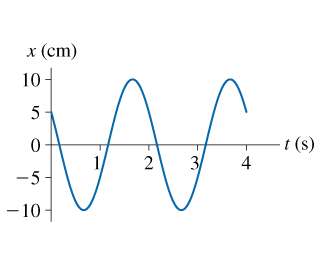# Problem: What is the frequency of the oscillation shown in the figure?

###### FREE Expert Solution

Frequency and periodic time are related as

$\overline{){\mathbf{f}}{\mathbf{=}}\frac{\mathbf{1}}{\mathbf{T}}}$

95% (45 ratings)###### Problem DetailsWhat is the frequency of the oscillation shown in the figure?